# GMAT Math : DSQ: Calculating the perimeter of a quadrilateral

## Example Questions

### Example Question #1 : Dsq: Calculating The Perimeter Of A Quadrilateral

What is the perimeter of Rhombus?

Statement 1: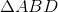has perimeter.

Statement 2: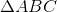is equilateral.

BOTH statements TOGETHER are sufficient to answer the question, but NEITHER statement ALONE is sufficient to answer the question.

EITHER statement ALONE is sufficient to answer the question.

Statement 1 ALONE is sufficient to answer the question, but Statement 2 ALONE is NOT sufficient to answer the question.

Statement 2 ALONE is sufficient to answer the question, but Statement 1 ALONE is NOT sufficient to answer the question.

BOTH statements TOGETHER are insufficient to answer the question.

BOTH statements TOGETHER are sufficient to answer the question, but NEITHER statement ALONE is sufficient to answer the question.

Explanation:

Each diagonal divides Rhombusinto two triangles, both isosceles.

Statement 1 alone establishes the perimeter of one such triangle. However, it does not make it clear what equal side lengths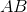and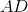and diagonal length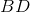are. For example,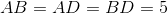fits the perimeter, but so does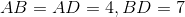.

Statement 2 alone gives no information about the actual lengths of the sides.

Assume both statements are true. Sinceis equilateral,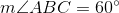. It follows that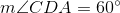, and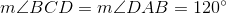. Also, the diagonals of a rhombus bisect their angles and are each other's perpendicular bisectors, so the rhombus, with their diagonals, is given below.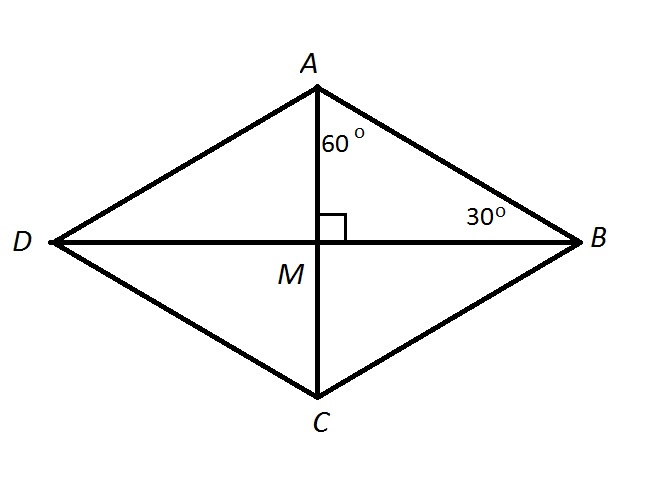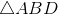has perimeter, which means that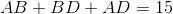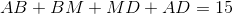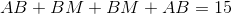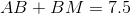Sinceis known to be a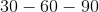triangle, the proportions of the side lengths are known; along with the above equation,, and, subsequently, the perimeter, can be determined.

### Example Question #71 : Geometry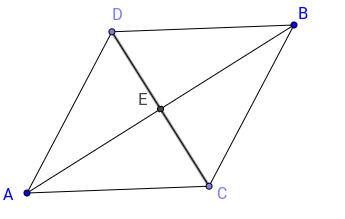What is the perimeter of quadrilateral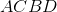(1) Diagonal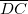and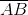are perpendicular with midpoint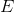.

(2)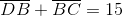Statements 1 and 2 together are not sufficient

Each statement alone is sufficient

Statement 2 alone is sufficient

Statement 1 alone is sufficient

Both statements together are sufficient

Both statements together are sufficient

Explanation:

To find the perimeter of the quadrilateral, we need to know whether it is of a special type of quadrilaterals and we need to know the length of the sides.

Statement 1 tells us only that the quadrilateral is a rhombus. Indeed, a quadrilateral with perpendicular diagonals intersecting at their midpoint must be a rhombus. However we don't know any length of the sides.

Statement 2 says gives us the length us two consecutive sides. It could be tempting to answer that it is sufficient, however, we can't conclude that the quadrilateral has equal lengths. Therefore this statement alone is insufficient.

Both statements together are sufficient since we can conclude that the quadrilateral is a rhombus, and twice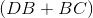will give us the perimeter.

### Example Question #8 : Other Quadrilaterals

Consider rectangle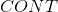.

I) Side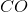is three fourths of side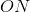.

II) Side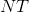is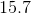meters long.

What is the perimeter of?

Statement II is sufficient to answer the question, but statement I is not sufficient to answer the question.

Statement I is sufficient to answer the question, but statement II is not sufficient to answer the question.

Either statement is sufficient to answer the question.

Both statements are needed to answer the question.

Both statements are needed to answer the question.

Explanation:

To find perimeter, we need to find the length of all the sides. Recall that rectangles are made up of two pairs of equal sides.

I) Relates one side to another non-equivalent side.

II) Gives us side, which must be equivalent to.

Use II) and I) to find all the side lengths, then add them up. Both are needed.

Recap:

Consider rectangle CONT

I) Side CO is three fourths of side ON

II) Side NT is 15.7 meters long

What is the perimeter of CONT?

Because we are dealing with a rectangle, we know the following: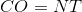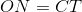Find perimeter with: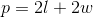Use I) and II) to write the following equation: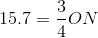So: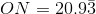And finally: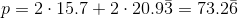### Example Question #31 : Quadrilaterals

Find the perimeter of the rectangle.

Statement 1:  The area of the rectangle is 24.

Statement 2:  The diagonal of the rectangle is 5.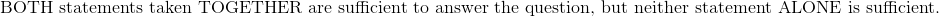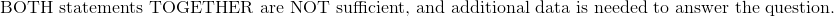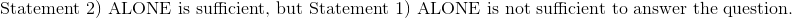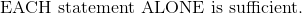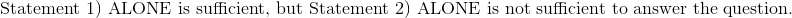Explanation:

Statement 1): The area of the rectangle is 24.

Write the area for a rectangle and substitute the value of the area.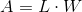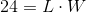The length and width of the rectangle are unknown, and each set of dimensions will provide a different perimeter.  This statement is insufficient to find the perimeter of the rectangle.

Statement 2): The diagonal of the rectangle is 5.

Given the diagonal of the rectangle, the Pythagorean Theorem can be used to solve for the diagonal.  Express the equation in terms of length and width.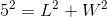Similar to the case in Statement 1), both the length and width are unknown, and the equation by itself is insufficient to solve for the perimeter of the rectangle.

Attempting to use both equations:andto solve for length and width will yield complex numbers as part of the solution.

Therefore:### All GMAT Math Resources